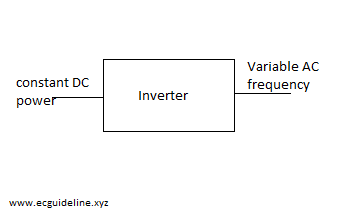## introduction D.C signal convert to A.C signal it is called inversion . and the device through which the process is edited that called inverter.Classification of inverters-

1. series  inverter.
2. parallel  inverter.
3. bridge inverters.

### 1. Series inverter-

It is inverter is called series because commutation components permanent connected in series with the load. series circuit must be under damped and current attain zero value due to nature  of series circuit.  it is also classified as self commutated inverter and load commutate inverter. It operate at high frequency 20 Hz to 100 GHz. three modes in series commutated  inverter.

#### Mode-1

The voltage DC voltage directly applies commutation components series circuit as soon as the SCR is turned on.  the polarity of capacitor  is show in the fig. B .

#### Mode - 2

the load current become zero from point a to b as the SCR1 turns off in this time period . the SCR1 and SCR2 are turns off in this time duration and voltage across capacitor becomes equal to DC voltage.

#### Mode-3

The SCR2 is turned on at point b due to it receives positive capacitor voltage the discharging of capacitor voltage. The discharge of capacitor is done through SCR2 and R-L circuit show in figure.

### Parallel inverter-

• This inverter even referred as center tapped transformer.
• capacitor is used for commutation.
• C provides complementary  commutation.

#### Mode-1

• Primary current flow from O to Q.
• By triggering SCR1,capacitor c will turn off SCR2.
• capacitor voltage A(+ve) w.r.t B plate.
• output is possitive.

#### Mode-2

• The primary current flow O to P.
• capacitor polarity that will change plate B w.r.t A plate.
• By triggering SCR2, capacitor will turn off SCR1.
• Output voltage is negative.